GATE Past Year Questions: Conduction

# GATE Past Year Questions: Conduction Notes | Study Heat Transfer - Mechanical Engineering

## Document Description: GATE Past Year Questions: Conduction for Mechanical Engineering 2022 is part of Steady State Heat Conduction for Heat Transfer preparation. The notes and questions for GATE Past Year Questions: Conduction have been prepared according to the Mechanical Engineering exam syllabus. Information about GATE Past Year Questions: Conduction covers topics like and GATE Past Year Questions: Conduction Example, for Mechanical Engineering 2022 Exam. Find important definitions, questions, notes, meanings, examples, exercises and tests below for GATE Past Year Questions: Conduction.

Introduction of GATE Past Year Questions: Conduction in English is available as part of our Heat Transfer for Mechanical Engineering & GATE Past Year Questions: Conduction in Hindi for Heat Transfer course. Download more important topics related with Steady State Heat Conduction, notes, lectures and mock test series for Mechanical Engineering Exam by signing up for free. Mechanical Engineering: GATE Past Year Questions: Conduction Notes | Study Heat Transfer - Mechanical Engineering
 1 Crore+ students have signed up on EduRev. Have you?

Question for GATE Past Year Questions: Conduction
Try yourself:Thermal conductivity is lower for



Question for GATE Past Year Questions: Conduction
Try yourself:Match the property with their units
Property
A. Bulk modulus
B. Thermal conductivity
C. Heat transfer coefficient
D. Heat flow rate

Units
1. W/s
2. N/m2
3. N/m3
4. W
5. W/mK
6. W/m2K

Codes: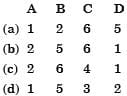Question for GATE Past Year Questions: Conduction
Try yourself:For a given heat flow and for the same thickness, the temperature drop across the material will be maximum for



Question for GATE Past Year Questions: Conduction
Try yourself:In descending order of magnitude, the thermal conductivity (a) Pure iron (b) Liquid water (c) Saturated water vapour (d) Pure aluminum can be arranged as



Question for GATE Past Year Questions: Conduction
Try yourself:As the temperature increases, the thermal conductivity of a gas



Question for GATE Past Year Questions: Conduction
Try yourself:Match Group with Group B:
Group A
A : Biot number
B : Grashoff number
C : Prandtl number
D : Reynolds number
Group B
1: Ratio of buoyancy to viscous force
2: Ratio of inertia force to viscous force
3: Ratio of momentum to thermal diffusivities
4: Ratio of internal thermal resistance to boundary layer thermal resistance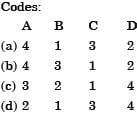Question for GATE Past Year Questions: Conduction
Try yourself:The temperature variation under steady heat conduction across a composite slab of two materials with thermal conductivities k1 and k2 is shown in figure then, which one of the following statements holds?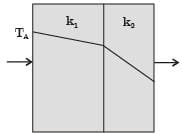Question for GATE Past Year Questions: Conduction
Try yourself:Heat is being transferred by convection from water at 48°C to a glass plate whose surface temp. is exposed to the water is at 40°C. The thermal conductivity of water is 0.6 W/mK and the thermal conductivity of glass is 1.2 W/mK.
The spatial gradient of temperature in the water at the water-glass interface is dT/dy = 1 × 104 K/m.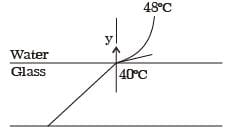The value of the temperature gradient in the glass at the water-glass interface in K/m is



Question for GATE Past Year Questions: Conduction
Try yourself:Heat is being transferred by convection from water at 48°C to a glass plate whose surface temp. is exposed to the water is at 40°C. The thermal conductivity of water is 0.6 W/mK and the thermal conductivity of glass is 1.2 W/mK.
The spatial gradient of temperature in the water at the water-glass interface is dT/dy = 1 × 104 K/m.The heat transfer coefficient h in w/m2K is



Question for GATE Past Year Questions: Conduction
Try yourself:One dimensional unsteady state heat transfer equation for a sphere with heat generation at the rate of q can be written



Question for GATE Past Year Questions: Conduction
Try yourself:A stainless steel tube (ks = 19 W/mK) of 2 cm ID and 5 cm OD is insulated with 3 cm thick asbestos (ka = 0.2 W/mK). If the temperature difference between the inner most and outermost surfaces is 600°C, the heat transfer rate per unit length is



Question for GATE Past Year Questions: Conduction
Try yourself:A well machined steel plate of thickness L is kept such that the wall temperature are Th and Tc as seen in the figure below. A smooth copper plate of the same thickness L is now attached to the steel plate without any gap as indicated in the figure below. The temperature at the interface is Tt. The temperatures of the outer walls are still the same at Th and Tc. The heat transfer rates are q1 and q2 per unit area in the two cases respectively in the direction shown. Which of the following statements is correct?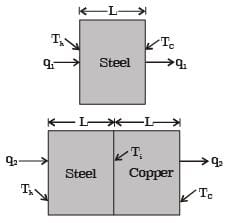Question for GATE Past Year Questions: Conduction
Try yourself:In a case of one dimensional heat conduction in a medium with constant properties, T is the temperature at position x, at time t. Then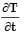is proportional to



Question for GATE Past Year Questions: Conduction
Try yourself:Heat flows through a composite slab, as shown below. The depth of the slab is 1 m. The k values are in W/mK. The overall thermal resistance in K/W is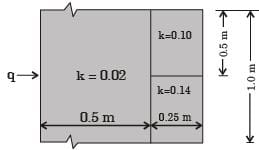Question for GATE Past Year Questions: Conduction
Try yourself:In a composite slab, the temperature at the interface (Tinter) between two materials is equal to the average of the temperature at the two ends. Assuming steady one dimensional heat conduction, which of the following statements is true about the respective thermal conductivities?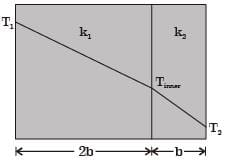Question for GATE Past Year Questions: Conduction
Try yourself:Heat is being transferred conductively from a cylindrical nuclear reactor fuel rod of 50 mm diameter to water at 75°C, under steady state condition, the rate of heat generation within the fuel element is 106 W/m3 and the convective heat transfer coefficient is 1 kW/m2K, the outer surface temperature of the fuel element would be



Question for GATE Past Year Questions: Conduction
Try yourself:Steady two-dimensional heat conduction takes place in the body shown in the figure below. The normal temperature gradients over surface P and Q can be considered to be uniform. The temperature gradient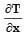at
surface Q is equal to 10 K/m. Surfaces P and Q are maintained at constant temperatures as shown in the figure, while the remaining part of the boundary is insulated. The body has a constant thermal conductivity of 0.1 W/mK. The values of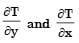at surface P are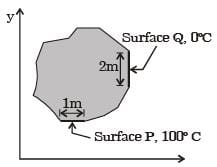Question for GATE Past Year Questions: Conduction
Try yourself:A brick wall (k = 0.9 W/mK) of thickness 0.18 m separates the warm air in a room from the cold ambient air. On a particular Winter day, the outside air temperature is - 5°C and the room needs to be maintained at 27°C. The heat transfer coefficient associated with outside air is 20 W/m2K. Neglecting the convective resistance of the air inside the room, the heat loss, in (W/m2), is



Question for GATE Past Year Questions: Conduction
Try yourself:A plastic sleeve of outer radius r0 = 1 mm covers a wire (radius r = 0.5 mm) carrying electric current, Thermal conductivity of the plastic is 0.12 W/mK. The heat transfer coefficient on the outer surface of the sleeve exposed to air is 25 W/m2K. Due to the addition of the plastic cover, the heat transfer from the wire to the ambient will



Question for GATE Past Year Questions: Conduction
Try yourself:One-dimensional steady state heat conduction takes place through a solid whose crosssectional area varies linearly in the direction of heat transfer. Assume there is no heat generation in the solid and the thermal conductivity of the material is constant and independent of temperature. The temperature distribution in the solid is



Question for GATE Past Year Questions: Conduction
Try yourself:A long glass cylinder of inner diameter = 0.03 m and outer diameter = 0.05 m carries hot fluid inside. If the thermal conductivity of glass = 1.05 W/mK, the thermal resistance (K/W) per unit length of the cylinder is



Question for GATE Past Year Questions: Conduction
Try yourself:Consider a long cylindrical tube of inner and outer radii, ri and r0, respectively, length L and thermal conductivity, k. Its inner and outer surfaces are maintained at Ti, and T0, respectively (Ti > T0). Assuming onedimensional steady state heat conduction in the radial direction, the thermal resistance in the wall of the tube is



Question for GATE Past Year Questions: Conduction
Try yourself:A hollow cylinder has length L, inner radius r1 outer radius r2, and thermal conductivity k. The thermal resistance of the cylinder for radial conduction is



Question for GATE Past Year Questions: Conduction
Try yourself:A slender rod of length L, diameterd (L >> d) and thermal conductivity k1 is joined with another rod of identical dimensions, but of thermal conductivit  k2, to form a composite cylindrical rod of length 2L. The heat transfer in radial direction and contact resistance are negligible. The effective thermal conductivity of the composite rod is



Question for GATE Past Year Questions: Conduction
Try yourself:For a current carrying wire of 20 mm diameter exposed to air (h = 20 W/m2K), maximum heat dissipation occurs when thickness of insulation (0.5 W/mK) is



Question for GATE Past Year Questions: Conduction
Try yourself:Two insulating material of thermal conductivity k and 2k are available for lagging a pipe carrying a hot fluid. If the radial thickness of each material is same



Question for GATE Past Year Questions: Conduction
Try yourself:It is proposed to coat a 1 mm diameter wire with enamel paint (k = 0.1 W/mK) to increase heat transfer with air. If the air side heat transfer coefficient is 100 W/m2K, the optimum thickness of enamel paint should be



Question for GATE Past Year Questions: Conduction
Try yourself:With an increase in the thickness of insulation around a circular pipe, heat loss to surroundings due to



Question for GATE Past Year Questions: Conduction
Try yourself:A 10 mm diameter electrical conductor is covered by an insulation of 2 mm thickness.The conductivity of the insulation is 0.08 W/mK and the convection coefficient at the insulation surface is 10 W/m2K. Addition of further insulation of the same material will



Question for GATE Past Year Questions: Conduction
Try yourself:Consider steady one-dimensional heat flow in a plate of 20 mm thickness with a uniform heat generation of 80 MW/m3. The left and right faces are kept at constant temperatures of 160°C and 120°C respectively. The plate has a constant thermal conductivity of 200 W/mK.
The location of maximum temperature within the plate from its left face is



Question for GATE Past Year Questions: Conduction
Try yourself:Consider steady one-dimensional heat flow in a plate of 20 mm thickness with a uniform heat generation of 80 MW/m3. The left and right faces are kept at constant temperatures of 160°C and 120°C respectively. The plate has a constant thermal conductivity of 200 W/mK.
The maximum temperature within the plate in °C is



Question for GATE Past Year Questions: Conduction
Try yourself:A coolant fluid at 30°C flows over a heated flat plate maintained at a constant temperature of 100°C. The boundary layer temperature distribution at a given location on the plate may be approximated as T= 30 + 70 expt(-y) where y(in m) is the distance normal to the plate and fis in °C. If thermal conductivity of the fluid is 1.0 W/mK, the local convective heat transfer coefficient (in W/m2K) at that location will be



Question for GATE Past Year Questions: Conduction
Try yourself:Consider one-dimensional steady state heat conduction, without heat generation, in a plane wall; with boundary conditions as shown in the figure below. The conductivity of the wall is given by k = k0 + bT; where k0 and b are positive constant, and T is temperature.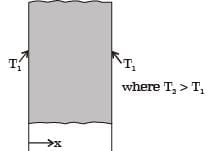As x increases, the temperature gradient (dT/dx) will



Question for GATE Past Year Questions: Conduction
Try yourself:Consider one-dimensional steady state heat conducting along x-axis (0 < x < L), through a plane wall with the boundary surfaces (x = 0 and x = L) maintained at temperature of 0°C and 100°C. Heat is generated uniformly through out the wall.Choose the CORRECT statement



Question for GATE Past Year Questions: Conduction
Try yourself:A cylindrical uranium fuel rod of radius 5 mm in a nuclear is generating heat at the rate of 4 × 107 W/m3. The rod is cooled by a liquid (convective heat transfer coefficient 1000 W/ m2K) at 25°C. At steady state, the surface temperature (in K) of the rod is



The document GATE Past Year Questions: Conduction Notes | Study Heat Transfer - Mechanical Engineering is a part of the Mechanical Engineering Course Heat Transfer.
All you need of Mechanical Engineering at this link: Mechanical Engineering

## Heat Transfer

58 videos|70 docs|85 tests
 Use Code STAYHOME200 and get INR 200 additional OFF

## Heat Transfer

58 videos|70 docs|85 tests

Track your progress, build streaks, highlight & save important lessons and more!

,

,

,

,

,

,

,

,

,

,

,

,

,

,

,

,

,

,

,

,

,

;